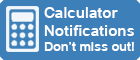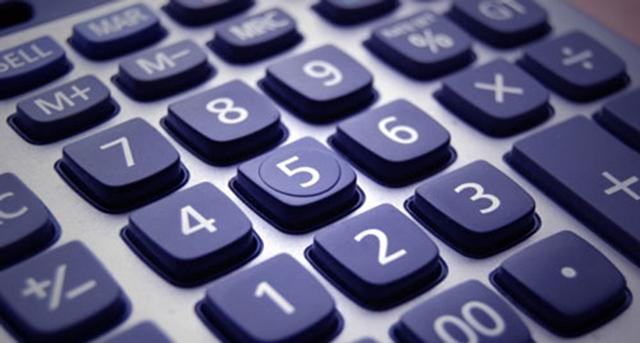Calculator Library
Free Online CalculatorsHome » 87 Calculators » Blog » Calculator Keys And Functions

Huge Domain Name Sale | Free Domains
Domain names on sale at crazy prices. Register a new domain for FREE when you buy another service.

## CALCULATOR KEYS AND FUNCTIONSCalculatorLibrary currently has 87 calculators online, and each calculator can have dozens of keys. Add to that all the real world calculators available and you'll soon realize there are a lot of calculator keys out there with a lot of different functions. What do all of these keys do?

Some calculator keys are obvious, but some are more obscure or specialized. Obviously we can't cover every possible key and function here, however this guide will try to explain the main ones you're likely to encounter.

Key Function

All Calculators
AC Clear - Clear all input from calculator
C or CLX Clear Display - Usually not memory
CE Clear Entry - Clear last entry
Del or Backspace Delete individual characters
0 1 2 3 4
5 6 7 8 9
Insert corresponding digit
. Insert decimal point
÷ Divide
x Multiply
- Subtract
± or CHS Change Sign - Positive or negative
% Calculate percentage of a number
M+ Memory Plus - Add value to memory
M- Memory Minus - Subtract value from memory
MS or STO Memory Store - Store value in memory
MC Memory Clear - Clear all memory
Enter or Return Enter the input
= Equals - Calculate the result

Scientific Calculators
⅟𝑥 Calculate reciprocal of a number
Calculate square root of a number
𝜋 Pi - The ratio of circle circumference to diameter is 3.1415926535 (10 decimals)
yx Raises number y to power x
Sin Sine - For Trigonometry functions
Cos Cosine - For Trigonometry functions
Tan Tangent - For Trigonometry functions
Asin Asine - Inverse sine of a number
Acos Acosine - Inverse cosine of a number
Atan Atangent - Inverse tangent of a number
Log Common Logarithm - Logarithm functions for base 10
Ln Natural Logarithm - Logarithm functions for base e
e Euler's number - Mathematical constant with value 2.7182818284 (10 decimals)
ex Natural Antilogarithm - Raises e to power x

Financial Calculators
EEX Enter Exponents
x⇄y Swap numbers in X & Y registers
12x Multiply displayed number by 12
12÷ Divide displayed number by 12
Percentage
∆% Percent Of Change between 2 numbers
%T Percent of Total
Calendar
D.MY Date Format - Day-month-year
M.DY Date Format - Month-day-year
DATE Change date by number of days
∆DYS Number of days between 2 dates
Time value of money
n Number of Payments
i Interest Rate per compounding period
PV Present Value - Initial cash flow
PMT Payment Amount
FV Future Value - Final cash flow
BEG Begin payment mode - compound interest
END End payment mode - compound interest
INT Calculate simple interest
AMORT Amortize no. of payments using PMT, i, PV
NPV Net Present Value of investment cash flows
IRR Internal Rate of Return (Yield) for above
PRICE Bond Price given desired yield to maturity
YTM Yield To Maturity given bond price
Depreciation
SL Straight-Line method
SOYD Sum-Of-the-Years-Digits method
DB Declining-Balance method
Statistics
Σ+ Adds statistics from X & Y registers
Σ- Cancels statistics from X & Y registers
s Standard Deviations using accumulated statistics

Programmable Calculators
R/S Run/Stop - Starts & stops stored programs
SST Single Step - Displays next program line
BST Back Step - Displays previous program line
GTO Go To - Go to program line number
PSE Pause - Pauses program and displays X register

Some calculators use "secondary" or "shift" keys, sometimes color coded such as f, g or 2nd that allow multiple functions to be allocated to each key. In other words, pressing a secondary key first changes the function processed by another key.

In addition to these widely used keys, there are often special keys that allow you to access unique functions for that particular calculator. Check the user manual or online instructions for more information about these unique or special function keys available on your chosen calculator.

Here at CalculatorLibrary, you can click or touch the keys on your screen to perform calculations using our free online calculators, however many calculators also have keyboard shortcuts that allow you to use your computer or device keyboard to enter many of the values. Check the "Notes" section near the bottom of each calculator to see these options.

 Previous Post« Graphing Calculators Next PostOnline Versus Real-World Calculators »